# Working with Cost Rate Tables

Similar to the ability to define a contoured resource availability, cost rate tables allow a project manager to model projected changes in a resource's rates over time, as well as allow a resource to have several different rate structures. This is useful when a resource's work is billed at different rates, depending on the type of work he does. For example, if a resource develops in Visual Basic, he is billed at \$150 per hour, but if he develops in C++, he is billed at \$175 per hour .

The "Defining Different Rates Over Time" section shows you how to define a changing rate over time. An example of this is when a project manager knows that a resource will receive a raise in pay on a specific date and she wants the project cost projections to include that raise.

#### Defining Different Rates Over Time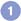Double-click on the resource for which you want to define costs.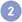Select the Costs tab.The " ”" in the first row means that the rates on this row are in effect for all dates up to the next row's Effective date.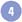\$75 is the Standard Rate that was entered for this resource in the Resource Sheet view.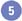Define a new Effective date by entering the date here (6/30).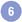On 6/30 we want our resource's Standard Rate to become \$85 per hour.Then on 12/31 we want our resource to get a 10% raise. Entering 10% in the Standard Rate field causes Project to calculate a 10% raise and enter that value when you change the field selection.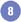In this example, from the beginning of time until 6/29 the resource's standard rate is \$75 per hour. Then on 6/30, it becomes \$85 per hour, and finally on 12/31 it becomes \$93.50 per hour.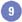Click OK.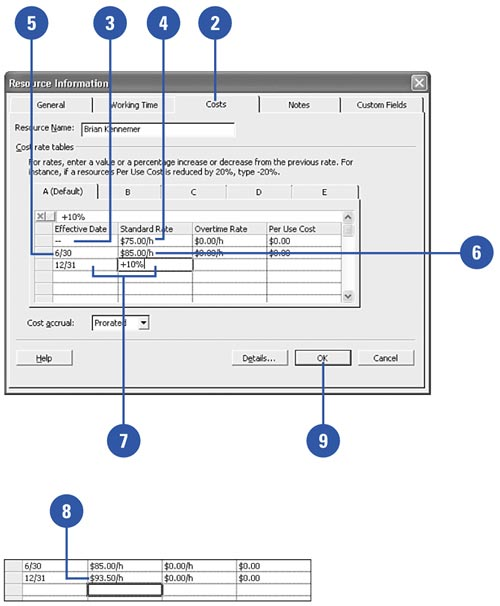#### Defining Different Rate TablesDouble-click on the resource for which you want to define costs.Select the Costs tab.Select one of the Rate Table tabs (B “E). Tab A is always the default rate table used when assigning resources.Enter the Standard Rate for this rate table, and/or edit the rate structure over time as needed.Click OK.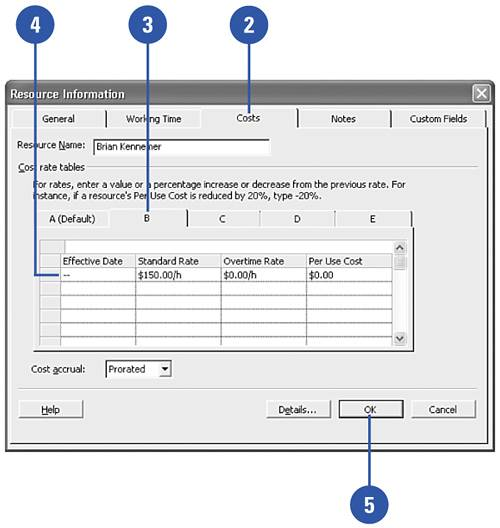Using cost rate tables when assigning resources will be covered in a later chapter.

#### Defining Resource Costs

The Standard Rate, Overtime Rate, and Per Use Cost for a resource is what Project uses to calculate the cost of using that resource to do the work on your tasks . Working with and understanding task costs are covered later.

Resource costs and how they affect a task's costs are made up of four basic elements (each of which are pointed out in the figure):

Standard RateThe rate Project uses to calculate the cost of regular (non-overtime) work on a task assignment.

Overtime RateThe rate Project uses to calculate the cost of overtime work on a task assignment.

Per Use CostA cost that Project adds to the cost of the task assignment, regardless of how much work is associated with the assignment.

Accrue AtThe method Project uses to calculate costs.

• Prorated means that costs are accrued according to the percentage of work that is complete on the task.

• Start means that all costs associated with the task assignment become Actual Costs as soon as work starts on the task.

• Finish means that none of the costs associated with the task will become Actual Costs until the task is 100% complete.

The default method is Prorated.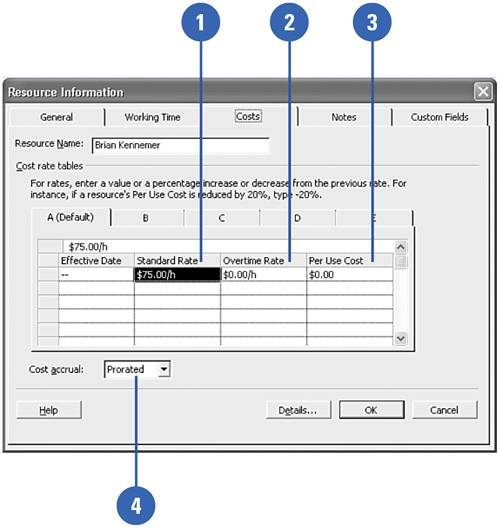Show Me Microsoft Office Project 2003
ISBN: 0789730693
EAN: 2147483647
Year: 2003
Pages: 204